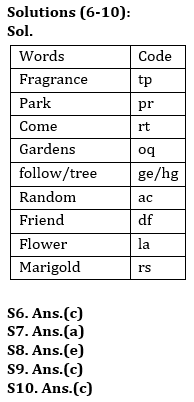Latest Banking jobs   »

# Reasoning Quiz For RBI Grade B Phase 1 2023-25th May

Directions (1-3): Study the following information carefully and answer the given questions.
Amongst five persons, P, R, S, T and U, each has different weight. P’s weight is more than R and T. S weight is less than U. Only two persons weight is more than P whose weight is 65kg. Youngest person weight is 55kg.

Q1. Who among the following is youngest person?
(a) U
(b) R
(c) S
(d) T
(e) Can’t be determined

Q2. If U’s weight is 70kg, then what may be the weight of S?
(a) 63
(b) 64
(c) 68
(d) 72
(e) None of these

Q3. How many persons are heavier than R?
(a) One
(b) Two
(c) Three
(d) None
(e) Can’t be determined

Q4. How many pairs of letters are there in the word ‘TRADITIONAL’, each of which have as many letters between them as they have in the English alphabet?
(a) More than Four
(b) One
(c) Three
(d) Two
(e) None

Q5. Adarsh ranked 25th from the top and 36th from the bottom in a class. How many students are there in the class?
(a) 61
(b) 59
(c) 52
(d) 58
(e) 60

Direction (6-10): Study the following information to answer the given questions:
In a certain code language
‘Random friend flower’ is written as ‘la ac df’,
‘Random fragrance marigold garden park’ is written as ‘oq pr rs ac tp’,
‘Follow friend fragrance marigold tree’ is written as ‘tp df rs ge hg’
‘Come fragrance park’ is written as ‘rt tp pr’.

Q6. What is the code for ‘park’?
(a) oq
(b) rs
(c) pr
(d) ac
(e) tp

Q7. ‘Come marigold fragrance garden’ could be coded as?
(a) tp rt rs oq
(b) rt ac rs ge
(c) df rt rs oq
(d) rt ac pr oq
(e) None of these

Q8. ‘hg’ stands for?
(a) Friend
(b) Fragrance
(c) Tree
(d) Follow
(e) Either ‘follow’ or ‘tree’

Q9. Which of the following may be the code for ‘keep fragrance flower’?
(a) tp la rt
(b) la df oq
(c) lb la tp
(d) ge la ac
(e) None of these

Q10. ‘tp oq pr’ is the code for?
(a) Marigold fragrance park
(b) Come fragrance park
(c) Fragrance garden park
(d) Can’t be determined
(e) None of these

Solutions

Solutions (1-3):
Sol. The arrangement will be: U > S > P > R/T > T/R

S1. Ans(e)
S2. Ans(c)
S3. Ans(e)

S4. Ans.(c)
Sol. LO, LN, NO

S5. Ans(e)
Sol. Number of students in the class= 25+36-1=60## FAQs

### How many sections are there in the RBI Grade B Phase 1 Exam?

There are 4 sections in the RBI Grade B Phase 1 Exam i.e. English Language, General Awareness, Quantitative Aptitude & Reasoning.

#### Congratulations!Union Budget 2023-24: Free PDF